Search Terms#Short Break

## Find the gain of the stack antenna

We may use antenna in a stack to send radio waves stronger and farther distance when operating in VHF and UHF especially. Stacking the antennas requires a large volume of space, but the gain of the antennas is greatly increased. Therefore, you can easily imagine that the radio wave is stronger and farther distance. This applies not only to transmission but also to reception, and even weak signals can be highlighted by using a stack antenna.

### Difference between single antenna and double row stack

The antenna in Figure 1 is a 10-element Yagi antenna in the 430 MHz band manufactured by Diamond Antenna. The model name is A430S10R2. The photo on the right shows the antenna on the left in a two-row stack.

According to the manufacturer's specifications, the antenna gain is stated as 13.1 dBi for a single antenna. (Quoted from the company's HP) The right is the one when the same antenna as the left is stacked in two rows. The gain of the two-row stack is stated in the company's specifications as 15.1 dBi. You can see that the 2-row stack increases by 2 dB.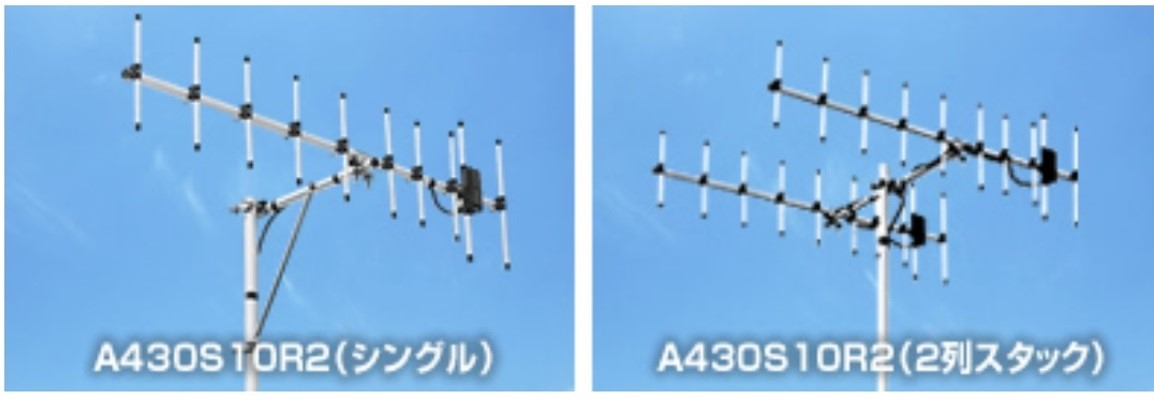Figure 1 Yagi antenna for the 430 MHz band (quoted from the company's website)

In addition, by changing the antenna from single to double stack, the beam width becomes sharper. The beam direction is sharper and it is possible to escape from interferences.Figure 2 Horizontal plane directivity of A430S10R2, Left: Single Right: 2-row stack

### Formula for calculating antenna gain for a two-row stack

If the gain G (dB) of a single antenna is stacked with n antennas, the gain Ga (dB) can be calculated by the formula below, although it is a theoretical value.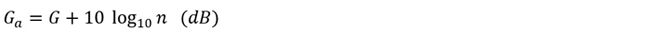### Let's use the formula to find the antenna gain of a two-row stack

Let's take the antenna of 10 element Yagi antenna shown in Figure 1 as an example. Substituting a numerical value into the formula shown above gives the following.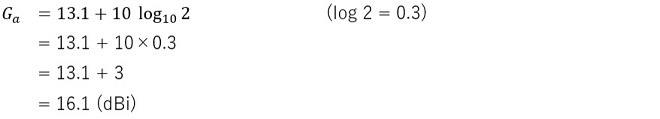As mentioned earlier, the gain of the single Yagi antenna is 13.1 dBi, which is stated on the company's HP. In this calculation, the gain was 16.1 dB for a two-row stack. By making it a stack, it has increased by 3 dB in the calculations.

The company's HP for this antenna states it as 15.1 dBi. There is a difference of 1 dB from the calculation. 15.1dBi is an actual measurement value, not a calculated value. Since it is an antenna used for actual communication, it is appreciated that the measured value is posted instead of the theoretical value.

The difference between the calculated value and the measured value is due to the fact that the stack distance and signal distributor loss that affect the antenna aperture are included in actual operation, and the calculated value is expected to increase the gain by 3 dB when stacked. However, in actual operation, it seems to be about 2 dB higher.

### Let's find the antenna gain of a 2-row 2-stage stack

Finally, let's find the gain of the antenna of the 2-row 2-stage stack as shown in the figure below. The formula for the calculation is the same as described above. The number of stages has also been increased, but the only difference is the value of n (= number of antenna). If you enter the numerical value in to the formula, it will be as follows.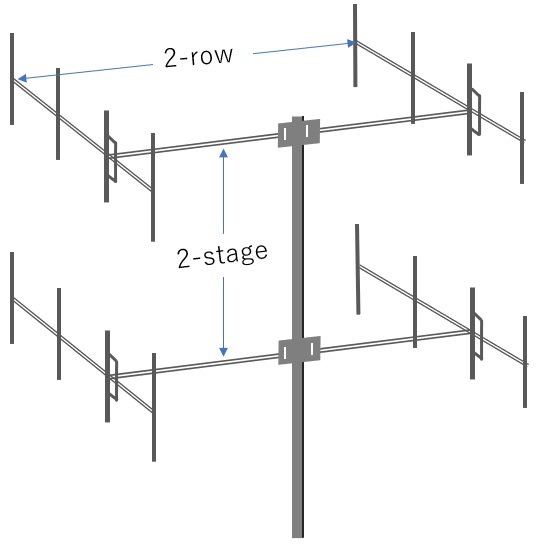Figure 3 4-element Yagi antenna 2-row, 2-stage stack

<Definition>
G: 7 dBi for the gain of the single Yagi antenna
n: Number of antenna connection in parallel
Ga: Stack antenna gain (dBi)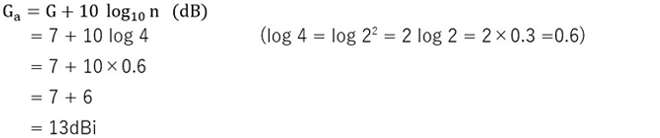### Summary

It was found that changing the antenna from a single to a two-row stack increases the gain by 3 dB regardless of the gain of the original antenna. Furthermore, if the two-row stack is made into two stages and a total of four single antennas are used as stack antennas as shown in Figure 3, it will be further increased by 3 dB, which means that the total increase is 6 dB from the original antenna.

In short, if the number of antennas is doubled, the gain will increase by 3dB. A two-row, two-stage stack using four single antennas had a gain of 13 dBi. If this is further doubled to 4 rows and 2 stages using 8 single antennas, it is 3 dB up, so 13 + 3 = 16 dBi.

CL